# 当我自己做了一个网页版的我的世界 ...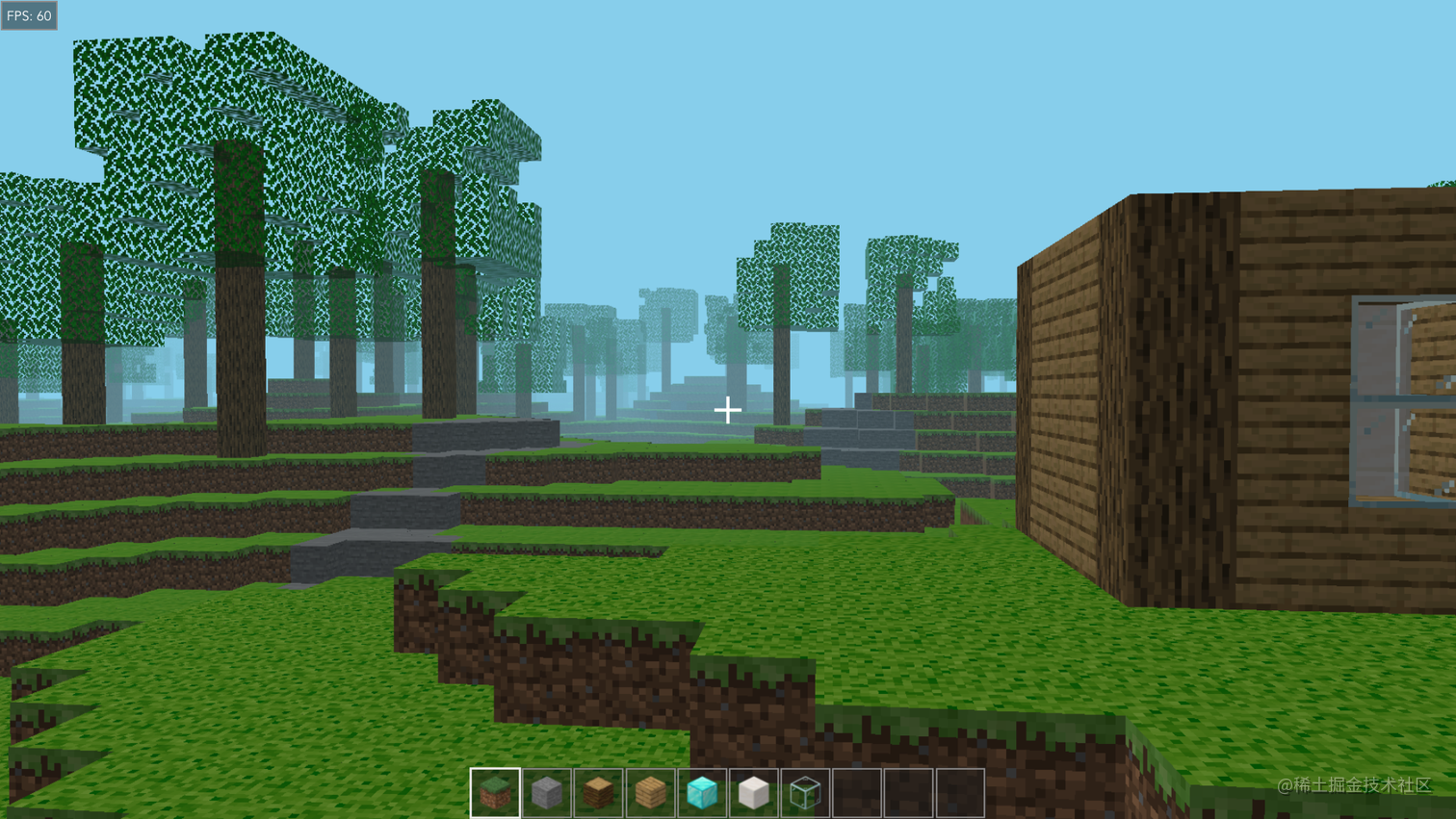GitHub 地址: github.com/vyse12138/m…

P.S. 目前还不支持手机端, 不过手机端的小伙伴可以先看看视频 :<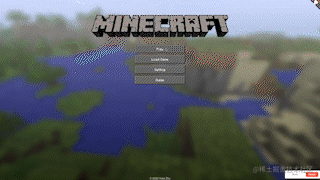## 介绍

### 游戏介绍

• 方块的放置 / 破坏
• 选择不同的方块类型
• 移动和碰撞检测
• 随机的地形和树木生成
• 无限的世界
• 保存 / 读取游戏
• 音效和背景音乐
• 可调节的渲染距离和视野范围
• 基本的 UI

• 生成水
• 更多的保存栏位
• 手机支持

### 玩法介绍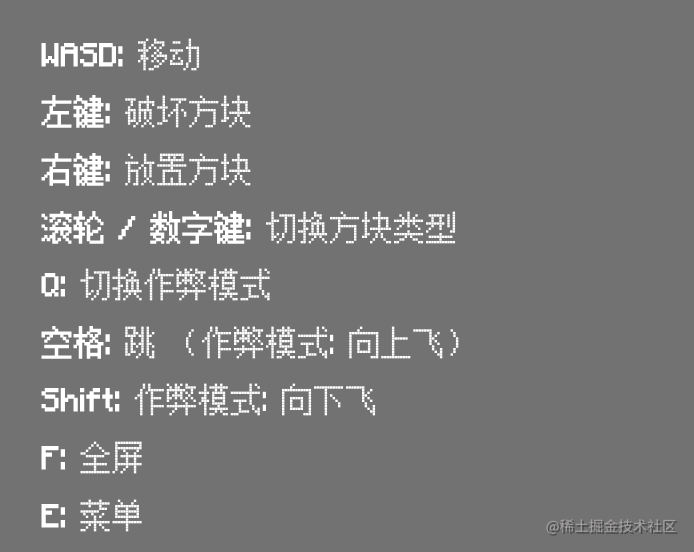### 源码介绍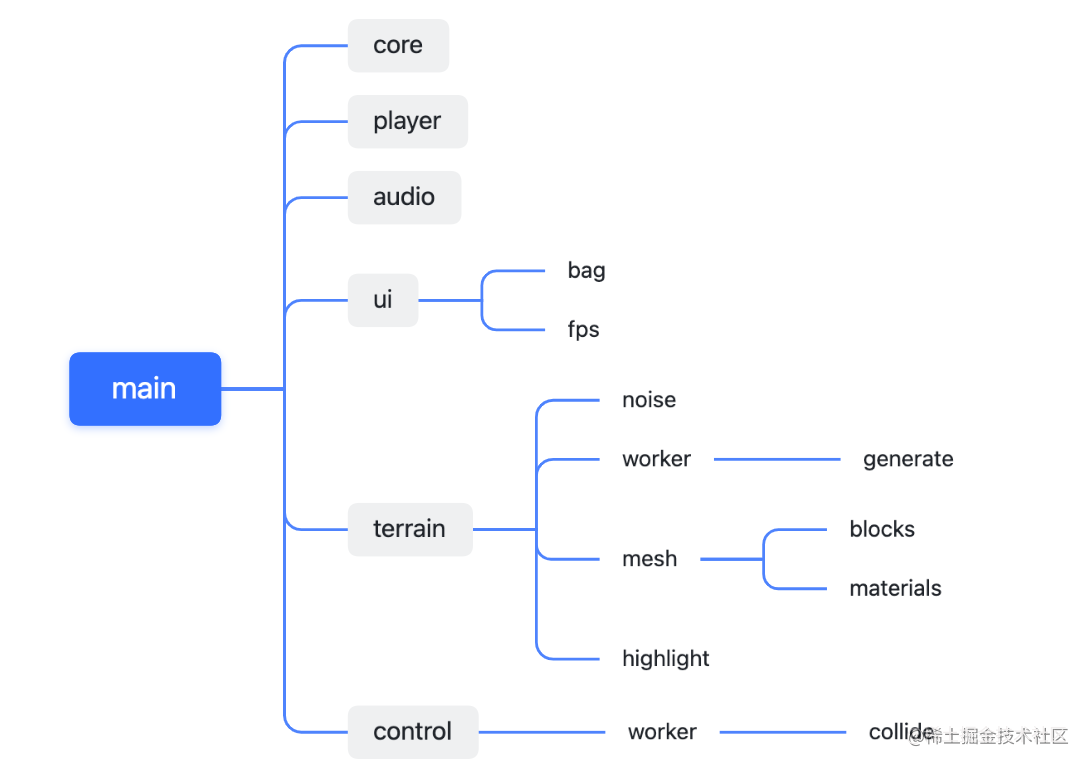• `Core`: 包含了 `Three.js` 中的一些核心内容, 并且进行一些初始化设定
• `Player`: 包含了玩家的一些基础属性以及当前模式(行走, 奔跑, 作弊等)
• `Audio`: 主要进行声音的导入, 并且暴露了一些用于播放音乐的 api
• `Terrain`: 包含了与地形相关的各种内容:
• `Noise`: 通过柏林噪音实现了地形, 树木, 方块类型的随机生成算法
• `GenerateWorker`: 通过 `web worker` 的方式实现了地形动态生成的算法
• `Mesh`: 场景中基础的网格体(方块)
• `Blocks`: 自定义方块类
• `Materials`: 各种方块的材质加载
• `Highlight`: 实现了实时高亮准心位置方块的算法
• `Control`: 包含了各种与操作相关的算法, 比如移动, 镜头转动, 碰撞检测等
• `CollideWorker`: 在 `web worker` 中实现碰撞检测以提高运行效率
• ui：包含了 ui 界面以及其功能:
• `Bag`: 放置不同方块的背包
• `FPS`: 实时展示当前 `fps`

## 核心技术点

### 随机的地形生成

``````import { ImprovedNoise } from 'three/examples/jsm/math/ImprovedNoise'

export default class Noise {
noise = new ImprovedNoise()
seed = Math.random()
stoneSeed = this.seed * 0.4
coalSeed = this.seed * 0.5
treeSeed = this.seed * 0.7
leafSeed = this.seed * 0.8

get = (x: number, y: number, z: number) => {
return this.noise.noise(x, y, z)
}
}

``````const blocks: THREE.InstancedMesh[] = []
blocks[i].instanceMatrix = new THREE.InstancedBufferAttribute(
new Float32Array(maxCount * blocksFactor[i] * 16),
16
)

``````
for (
let x = -chunkSize * distance + chunkSize * chunk.x;
x < chunkSize * distance + chunkSize + chunkSize * chunk.x;
x++
) {
for (
let z = -chunkSize * distance + chunkSize * chunk.y;
z < chunkSize * distance + chunkSize + chunkSize * chunk.y;
z++
)
const yOffset = Math.floor(
noise.get(x / noise.gap, z / noise.gap, noise.seed) * noise.amp
)
matrix.setPosition(x, y + yOffset, z)
// 如果为草方块
blocks[BlockType.grass].setMatrixAt(
blocksCount[BlockType.grass]++,
matrix
)
// 如果为其他方块
...
}
}

#### 无限动态地形生成

``````  update = () => {
this.chunk.set(
Math.floor(this.camera.position.x / this.chunkSize),
Math.floor(this.camera.position.z / this.chunkSize)
)

// 当进入新的区块时, 触发一次渲染
if (
this.chunk.x !== this.previousChunk.x ||
this.chunk.y !== this.previousChunk.y
) {
this.generate()
}

this.previousChunk.copy(this.chunk)
}

``````  // 将数据传入 web worker
generate = () => {
this.blocksCount = new Array(this.blocks.length).fill(0)
this.generateWorker.postMessage({
distance: this.distance,
chunk: this.chunk,
noiseSeed: this.noise.seed,
treeSeed: this.noise.treeSeed,
stoneSeed: this.noise.stoneSeed,
coalSeed: this.noise.coalSeed,
idMap: new Map<string, number>(),
blocksFactor: this.blocksFactor,
blocksCount: this.blocksCount,
customBlocks: this.customBlocks,
chunkSize: this.chunkSize
})
}

// 处理返回的数据
this.generateWorker.onmessage = (
msg: MessageEvent<{
idMap: Map<string, number>
arrays: ArrayLike<number>[]
blocksCount: number[]
}>
) => {
this.resetBlocks()
this.idMap = msg.data.idMap
this.blocksCount = msg.data.blocksCount

for (let i = 0; i < msg.data.arrays.length; i++) {
this.blocks[i].instanceMatrix = new THREE.InstancedBufferAttribute(
(this.blocks[i].instanceMatrix.array = msg.data.arrays[i]),
16
)
}

for (const block of this.blocks) {
block.instanceMatrix.needsUpdate = true
}
}

#### 无限动态地形生成(垂直方向)

``````  generateAdjacentBlocks = (position: THREE.Vector3) => {
const { x, y, z } = position
const noise = this.noise
const yOffset = Math.floor(
noise.get(x / noise.gap, z / noise.gap, noise.seed) * noise.amp
)

if (y > 30 + yOffset) {
return
}

// 生成周围的方块
this.buildBlock(new THREE.Vector3(x, y - 1, z), type)
this.buildBlock(new THREE.Vector3(x, y + 1, z), type)
this.buildBlock(new THREE.Vector3(x - 1, y, z), type)
this.buildBlock(new THREE.Vector3(x + 1, y, z), type)
this.buildBlock(new THREE.Vector3(x, y, z - 1), type)
this.buildBlock(new THREE.Vector3(x, y, z + 1), type)

this.blocks[type].instanceMatrix.needsUpdate = true
}

### 视角移动 / 玩家移动

• 视角移动 (移动鼠标)
• 玩家移动 (WASD 键和空格等)

#### 玩家移动

``````  setMovementHandler = (e: KeyboardEvent) => {
if (e.repeat) {
return
}
switch (e.key) {
case 'w':
case 'W':
this.velocity.x += this.player.speed
break
case 's':
case 'S':
this.velocity.x -= this.player.speed
break
case 'a':
case 'A':
this.velocity.z -= this.player.speed
break
case 'd':
case 'D':
this.velocity.z += this.player.speed
break
}
}

update = () => {
// 更新位置, delta 为时间因子
this.control.moveForward(this.velocity.x * delta)
this.control.moveRight(this.velocity.z * delta)
}

#### 跳跃, 重力, 和地面检测

``````  setMovementHandler = (e: KeyboardEvent) => {
if (e.repeat) {
return
}
switch (e.key) {
case ' ':
if (this.player.mode === Mode.walking) {
// 如果当前不是跳跃状态, 进行一次跳跃
// 并暂时取消地面的碰撞检测(否则会跳不起来 =.=)
if (!this.isJumping) {
this.velocity.y = 8
this.isJumping = true
this.downCollide = false
this.far = 0
setTimeout(() => {
this.far = this.player.body.height
}, 300)
}
}
break
}
}
update = () => {
// 重力
if (Math.abs(this.velocity.y) < this.player.fallingSpeed) {
this.velocity.y -= 25 * delta
}

// downCollide = 是否触碰到地面, 具体的实现会在下面介绍
// 如果碰到地面, 则清零下落速度, 并且更新更新为可跳跃状态
if (this.downCollide && !this.isJumping) {
this.velocity.y = 0
} else if (this.downCollide && this.isJumping) {
this.isJumping = false
}
}

#### 碰撞检测

``````  collideCheck = (
side: Side,
position: THREE.Vector3,
noise: Noise,
customBlocks: Block[],
far: number = this.player.body.width
) => {
const matrix = new THREE.Matrix4()

// 重置模拟方块
let index = 0
this.tempMesh.instanceMatrix = new THREE.InstancedBufferAttribute(
new Float32Array(100 * 16),
2
)

// 获取方块位置
let x = Math.round(position.x)
let z = Math.round(position.z)
let y =
Math.floor(
noise.get(x / noise.gap, z / noise.gap, noise.seed) * noise.amp
) + 30

// 下方的碰撞检测
switch (side) {
case Side.down:
this.raycasterDown.ray.origin = position
this.raycasterDown.far = far
break
}

// 更新模拟方块
matrix.setPosition(x, y, z)
this.tempMesh.setMatrixAt(index++, matrix)
this.tempMesh.instanceMatrix.needsUpdate = true

// 更新 downCollide, 即判断否触碰到地面
const origin = new THREE.Vector3(position.x, position.y - 1, position.z)
switch (side) {
case Side.down: {
const c1 = this.raycasterDown.intersectObject(this.tempMesh).length
c1 ? (this.downCollide = true) : (this.downCollide = false)
break
}
}

#### 又到了玩家移动

``````  // 计算出镜头的朝向
let vector = new THREE.Vector3(0, 0, -1).applyQuaternion(
this.camera.quaternion
)
let direction = Math.atan2(vector.x, vector.z)

// 镜头朝向场景中的正 x 轴方向, 偏移量为 +45 度到 -45 度之间
if (direction < Math.PI && direction > 0 && this.velocity.x > 0) {
if (
// 镜头和方块有夹角时的左右滑动
(!this.leftCollide && direction > Math.PI / 2) ||
(!this.rightCollide && direction < Math.PI / 2)
) {
this.moveZ(Math.PI / 2 - direction, delta)
}
} else if (
!this.leftCollide &&
!this.rightCollide &&
this.velocity.x > 0
) {
// 若前方无阻当, 则直接前进
this.control.moveForward(this.velocity.x * delta)
}

### 方块放置 / 破坏

#### 准星高亮

``````  update() {
// 重置上一次的高亮
this.scene.remove(this.mesh)
this.index = 0
this.instanceMesh.instanceMatrix = new THREE.InstancedBufferAttribute(
new Float32Array(1000 * 16),
16
)

const position = this.camera.position
const matrix = new THREE.Matrix4()
const noise = this.terrain.noise

let xPos = Math.round(position.x)
let zPos = Math.round(position.z)

// 模拟周围一圈的方块
for (let i = -8; i < 8; i++) {
for (let j = -8; j < 8; j++) {
let x = xPos + i
let z = zPos + j
let y =
Math.floor(
noise.get(x / noise.gap, z / noise.gap, noise.seed) * noise.amp
) + 30
matrix.setPosition(x, y, z)
this.instanceMesh.setMatrixAt(this.index++, matrix)
}
}

// 高亮新的方块
this.raycaster.setFromCamera({ x: 0, y: 0 }, this.camera)
this.block = this.raycaster.intersectObject(this.instanceMesh)
if (
this.block &&
this.block.object instanceof THREE.InstancedMesh &&
typeof this.block.instanceId === 'number'
) {
this.mesh = new THREE.Mesh(this.geometry, this.material)
let matrix = new THREE.Matrix4()
this.block.object.getMatrixAt(this.block.instanceId, matrix)
const position = new THREE.Vector3().setFromMatrixPosition(matrix)

this.mesh.position.set(position.x, position.y, position.z)
}
}

#### 方块放置 / 破坏

• 如果为破坏, 则删除高亮方块
• 如果为放置, 则在高亮方块对应的面上放置一个新的方块

``````clickHandler = (e: MouseEvent) => {
e.preventDefault()
this.raycaster.setFromCamera({ x: 0, y: 0 }, this.camera)
const block = this.raycaster.intersectObjects(this.terrain.blocks)
const matrix = new THREE.Matrix4()

switch (e.button) {
// 左键破坏
case 0:
{
if (block && block.object instanceof THREE.InstancedMesh) {
block.object.getMatrixAt(block.instanceId!, matrix)
const position = new THREE.Vector3().setFromMatrixPosition(matrix)

// 移除方块
block.object.setMatrixAt(
block.instanceId!,
new THREE.Matrix4().set(...new Array(16).fill(0))
)
block.object.instanceMatrix.needsUpdate = true

// 播放音效
this.audio.playSound(
BlockType[block.object.name as any] as unknown as BlockType
)

// 生成周围方块 (详见本文前面的垂直方向无限地形部分)
}
}
break
// 右键放置
case 2:
{
if (block && block.object instanceof THREE.InstancedMesh) {
// 计算新方块的位置
const normal = block.face!.normal
block.object.getMatrixAt(block.instanceId!, matrix)
const position = new THREE.Vector3().setFromMatrixPosition(matrix)

// 若已有重叠方块则直接返回
if (
position.x + normal.x === Math.round(this.camera.position.x) &&
position.z + normal.z === Math.round(this.camera.position.z) &&
(position.y + normal.y === Math.round(this.camera.position.y) ||
position.y + normal.y ===
Math.round(this.camera.position.y - 1))
) {
return
}

// 放置方块
matrix.setPosition(
normal.x + position.x,
normal.y + position.y,
normal.z + position.z
)
this.terrain.blocks[this.holdingBlock].setMatrixAt(
this.terrain.getCount(this.holdingBlock),
matrix
)
this.terrain.setCount(this.holdingBlock)

// 播放音效
this.audio.playSound(this.holdingBlock)

this.terrain.blocks[this.holdingBlock].instanceMatrix.needsUpdate =
true
}
}
break
}
}

### 保存 / 读取功能

``````export default class Block {
constructor(
x: number,
y: number,
z: number,
type: BlockType,
placed: boolean
) {
this.x = x
this.y = y
this.z = z
this.type = type
this.placed = placed
}
x: number
y: number
z: number
type: BlockType
placed: boolean
}

• 在我们放置方块时, 就会新增一个对应 `BlockType`的, `placed = true` 的方块
• 而在我们破坏方块时, 就会新增一个 `placed = false` 的方块

### 基本的 UI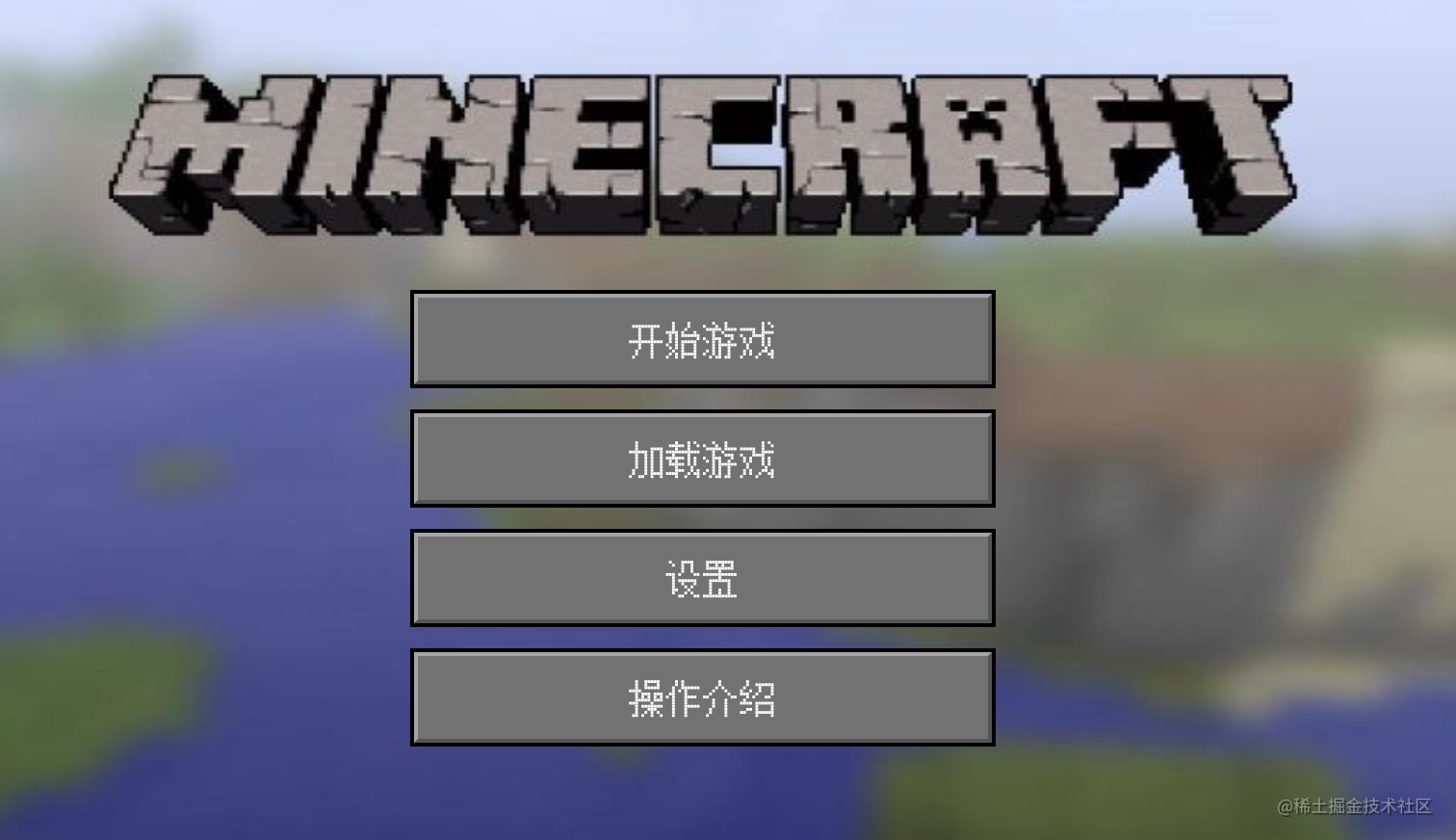## 最后

GitHub 地址: github.com/vyse12138/m…

•••• dragonir
8月前• kenshin
9月前••